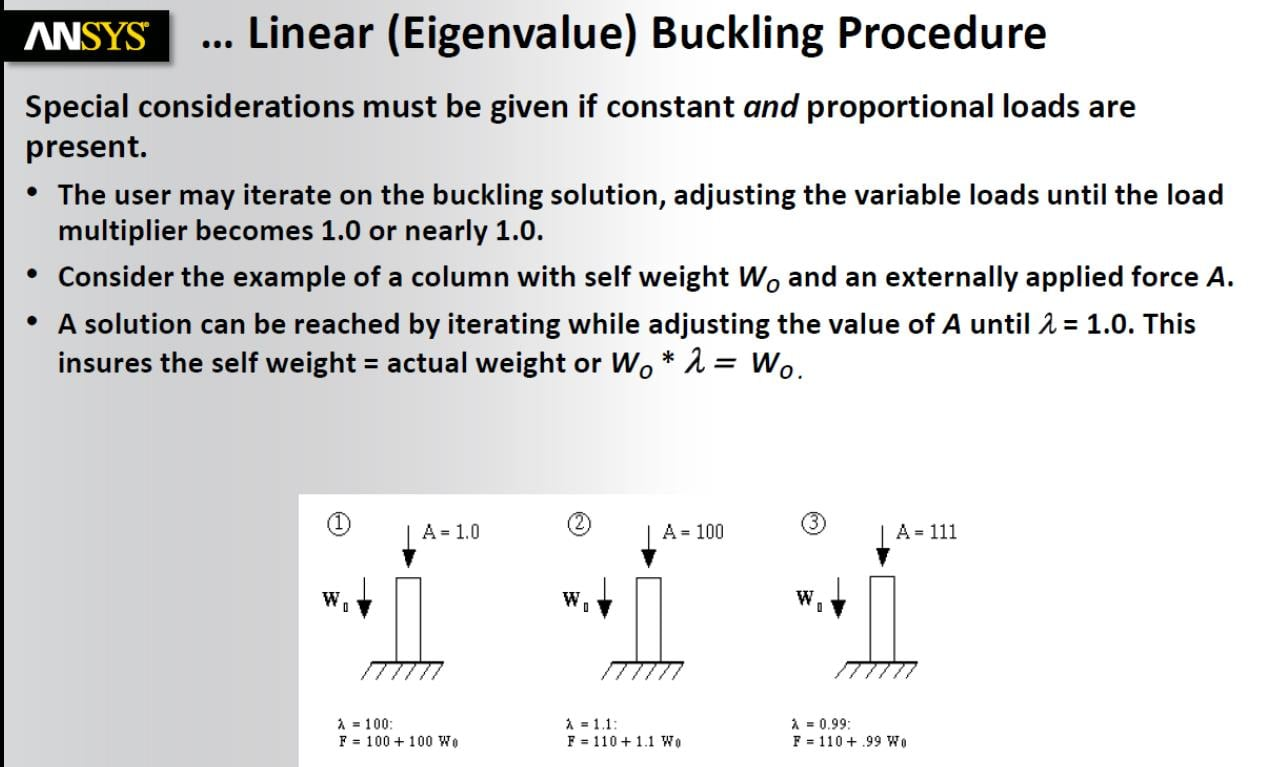# Linear eigenvalue buckling procedure - Constant and proportional (=variable) loads

Hello everyone,

I would like to understand how come the value of the variable load A is 1.0 at the first iteration in the following slide, and why lambda=100.

I know only that the goal is to adjust the variable load so that lambda=1; in this way I ensure that lambda does not affect the constant load W0, in fact, if lamda=1, I have W0*lambda=W0*1=W0.• A = 1.0 in the first iteration because users will often apply a unit load to a structure to solve the Linear Buckling problem. The reason they do that is when the solution provides a value of lamda, it is very easy to know the buckling load because the buckling load is A*lambda.

When there is self weight there are two items causing buckling, the applied load and the self weight. You want to know the applied load that is required to buckle without changing the weight, but the whole analysis is proportional so the lamda in the solution must multiply the applied load AND the weight. But you are not interested in when a heavier column would buckle, but this column.  So you must therefore adjust the applied load until lamba equals 1.0 then you have found the buckling load for that column weight.

• edited November 2019

Hello peteroznewman,

thank you so much for your clarification. There is still an unclear point: how do I know that the column buckles when A=111 and lambda=0.99? Why it cannot buckle when A=1 and lamda=0.99? Maybe the linear eigenvalue procedure tells me that lamba=0.99 if and only if A=111?

• Lamda is the solution. It tells you the buckling load is lamba*A.   If it takes A=111 to buckle then lamda = 1.0 in the solution.  If you put A = 1.0 then lamba will be 111 in the solution, for a problem where you can ignore the self-weight such as a horizontal column.

• edited November 2019

Perfect explanation. Thank you a lot peterroznewman.

• edited November 2019

Hello peteroznewman, sorry for the nuisance. I'd like to ask you if there is an example (video or at least documentation or book) where i can see the various steps in order to set in the right way the model and to read in the right way the results in the case in which there is a constant and a variable loads?

I did an example here.

Model: parallelepiped (height=1000 mm, square section with side=50 mm).

Boundary conditions: fixed support at base, standard earth gravity, 1 N  compressive load parallel to self weight load.

In this case I get a load multiplier=4188.6. Why? Ansys should implement the above iterative procedure to give me an unit load multiplier.